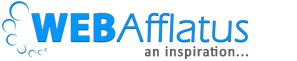# Mu Sigma Placement Papers – Aptitude Test Questions & SolutionsWe had Mu Sigma in our campus on 25th July 2013 here in IIITM Gwalior. Actually they had given contract to Cocubes.com and a team of 2 guys from cocubes.com came to our campus to conduct the test. I am going to give a brief scenario of the test. Will give an idea about the paper and the complexity of the paper. Visit also – Mu Sigma Group Discussion, Video Synthesis and Interview Round and Tips for Mu-Sigma GD. How to face Mu Sigma Group Discussion Round & watch video.

In first sight the paper looked tough. We had 20 questions and 30 minutes. A timer was there on the web page. So you can always count down your remaining time, which makes students more nervous. The question paper was divided into 3 sections. 8 Questions for basic Aptitudes, 7 from Data Interpretation and 5 from C Programing. In my paper, most of the questions were from Time- Distance, Time – Work, Probability, and Permutation. +1 for each question and 0.5 negative marks for each wrong answer. I would suggest that do not attempt all questions. Try to do 12-15 questions but correctly. That enough.
Questions for Aptitudes:
1. In a bag, there are 8 blue balls and 6 red. 2 balls are picked up without replacement. So what is the probability of picking red ball in 2nd attempt knowing that a blue ball has already been extracted?
2. There is 100 kg of food for 25 people, which will end in 2 days. So how many days it will take to finish 200 kg, if there are 30 people? (Not exact words but sense is similar. Answer was very confusing. Comment below the answer if you get it. Its : 31/3)
Question from Data Interpretation:
There were 7 questions. All easy but calculation was there. Sample questions:
There is a university which has total of 20,334 UG and 11,232 PG students. Below is a diagram showing % of UG students in various branch. Branches are R, S, T and V.Visit also – Mu Sigma Group Discussion, Video Synthesis and Interview Round
1. What is the ration of UG students in R and UG students in T.?
2. What is difference between UG R students and PG S students? (PG chart was also given)
Another question: There was a Bar chart table and questions were put from that chart. All easy just calculation.
3rd part was C programing. There were 5 questions. If you have basic knowledge of C, you can crack it. All questions from Loop, Array, and String.
Sample Questions.
1. What is the output if X=8;
Int main ()
{
y=1;
while (y<=x)
{
y=y*2
}
}
2. Which state is correct for the statement Array Ar = {3,3,3}
a. Ar  will be 3.
b. Ar  will be 3.
c. Ar  is the last element.
d. None of the these.
There were 3 more questions which I can’t remember but they were having only error. One C programing questions was having a statement n+ (it should be n++). So in that case the answer would be ‘No Output’.
Read more about – Mu Sigma Group Discussion, Video Synthesis and Interview Round and Tips for Mu-Sigma GD. How to face Mu Sigma Group Discussion Round & watch video.